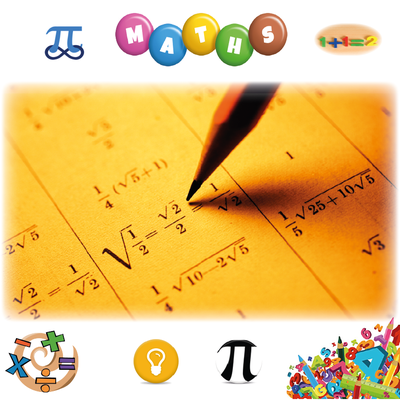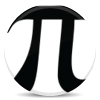Learn ﻿﻿﻿Mathematics

An easy way to learn Mathematics online for free. Learn Maths Basics & Prealgebra; Geometry, Algebra & Trigonometry; Precalculus, Calculus & much more through this very simple course. Multiple Choice Tests. Thanks to Mem creators, Contributors & Users.
65.4k учеников 286h средняя продолжительность100 x 100  Multiplication Table

30.3k 81hMaths GCSE

16.6k 50mMaths

Learn Times Tables

16.3k 1hMultiplication Tables

14k 2hMaths

Learn ﻿﻿﻿﻿﻿﻿﻿Statistics

12k 23h'Basic Trigonometric Identities'

11.9k 35mProbability Theory and Statistics (Introduction)

6.85k 7h100 Perfect Squares

6.19k 2hA level Edexcel Maths and Further Maths

6.17k 2h150 Digits of Pi

5.42k 37mEdexcel D1 Definitions

4.85k 25mMaths

General Maths (Methods) CAS VCE - Trigonometry

3.74k 21mMaths

1 - 144 Times Tables! Multiplication and division

3.65k 5hMaths

Conversions

3.25k 19m3.11k 22m